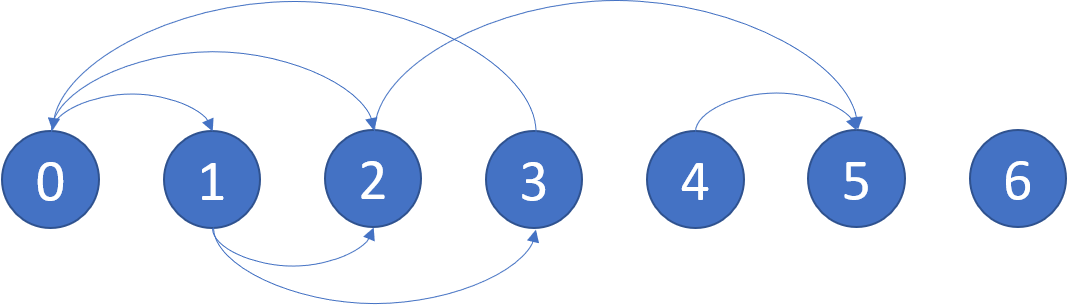802. Find Eventual Safe States

We start at some node in a directed graph, and every turn, we walk along a directed edge of the graph. If we reach a terminal node (that is, it has no outgoing directed edges), we stop.

We define a starting node to be safe if we must eventually walk to a terminal node. More specifically, there is a natural number `k`, so that we must have stopped at a terminal node in less than `k` steps for any choice of where to walk.

Return an array containing all the safe nodes of the graph. The answer should be sorted in ascending order.

The directed graph has `n` nodes with labels from `0` to `n - 1`, where `n` is the length of `graph`. The graph is given in the following form: `graph[i]` is a list of labels `j` such that `(i, j)` is a directed edge of the graph, going from node `i` to node `j`.

Example 1:```Input: graph = [[1,2],[2,3],,,,[],[]]
Output: [2,4,5,6]
Explanation: The given graph is shown above.
```

Example 2:

```Input: graph = [[1,2,3,4],[1,2],[3,4],[0,4],[]]
Output: 
```

Constraints:

• `n == graph.length`
• `1 <= n <= 104`
• `0 <= graph[i].legnth <= n`
• `graph[i]` is sorted in a strictly increasing order.
• The graph may contain self-loops.
• The number of edges in the graph will be in the range `[1, 4 * 104]`.

802. Find Eventual Safe States
``````struct Solution;

impl Solution {
fn eventual_safe_nodes(graph: Vec<Vec<i32>>) -> Vec<i32> {
let n = graph.len();
let mut res = vec![];
let mut state = vec![0; n];
for i in 0..n {
if Self::dfs(i, &mut state, &graph) {
res.push(i as i32);
}
}
res
}

fn dfs(u: usize, state: &mut [i32], graph: &[Vec<i32>]) -> bool {
match state[u] {
3 => false,
2 => true,
1 => {
state[u] = 3;
false
}
_ => {
state[u] = 1;
let mut s = 2;
for &v in &graph[u] {
if !Self::dfs(v as usize, state, graph) {
s = 3;
}
}
state[u] = s;
state[u] == 2
}
}
}
}

#[test]
fn test() {
let graph = vec_vec_i32![[1, 2], [2, 3], , , , [], []];
let res = vec![2, 4, 5, 6];
assert_eq!(Solution::eventual_safe_nodes(graph), res);
}
``````